# A) B) C) C) Enter electrons as e. A voltaic cell is constructed in which the...

A)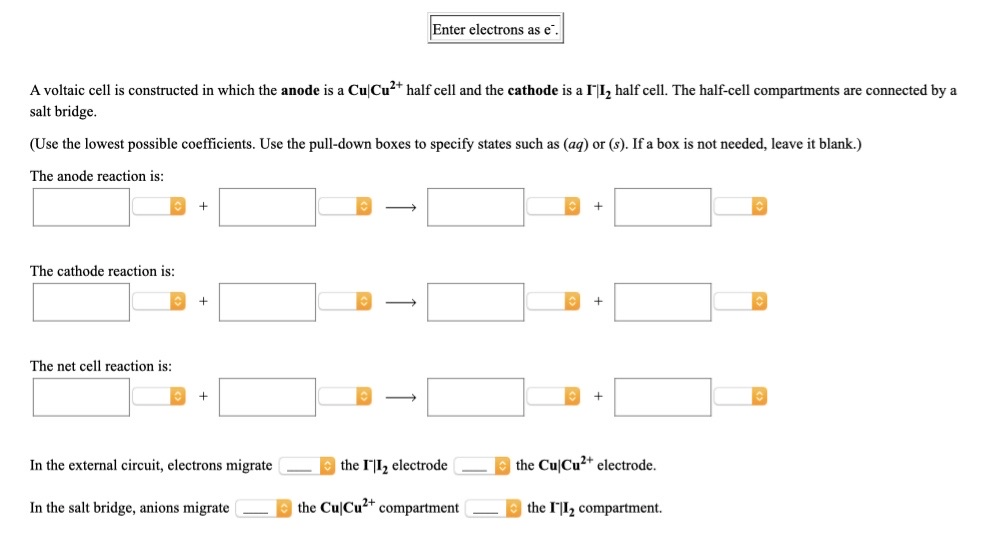B)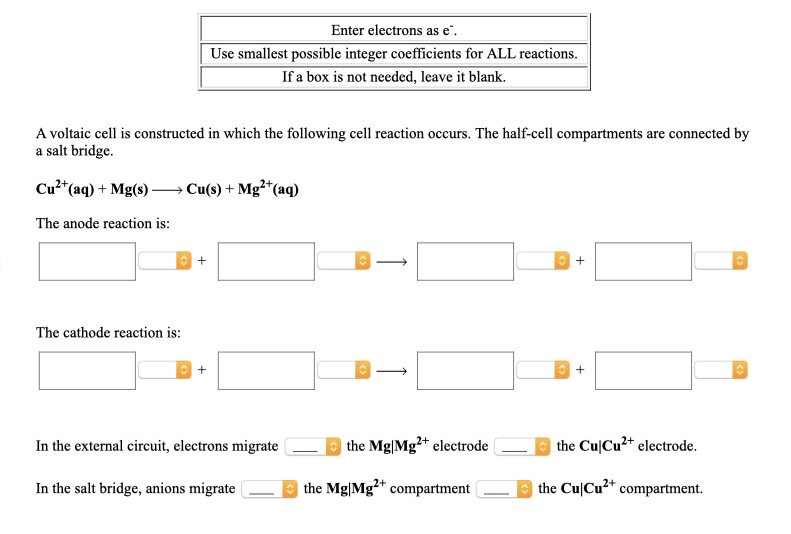C)

C)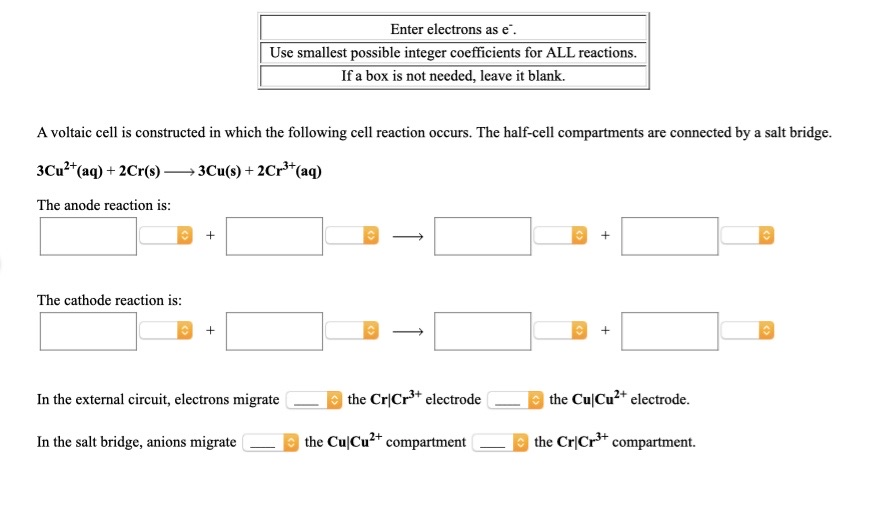Enter electrons as e. A voltaic cell is constructed in which the anode is a CuCu2+ half cell and the cathode is a II, half cell. The half-cell compartments are connected by a salt bridge. (Use the lowest possible coefficients. Use the pull-down boxes to specify states such as (aq) or (s). If a box is not needed, leave it blank.) The anode reaction is: The cathode reaction is: The net cell reaction is: In the external circuit, electrons migrate the "12 electrode the CuCu2+ electrode. In the salt bridge, anions migrate the CuCu2+ compartment - the 1"|I2 compartment.
Enter electrons as e'. | Use smallest possible integer coefficients for ALL reactions. If a box is not needed, leave it blank. A voltaic cell is constructed in which the following cell reaction occurs. The half-cell compartments are connected by a salt bridge. Cu2+ (aq) + Mg(s) —Cu(s) + Mg2+(aq) The anode reaction is: The cathode reaction is: In the external circuit, electrons migrate the Mg|Mg2+ electrode — the Cu|Cu2+ electrode. In the salt bridge, anions migrate the Mg|Mg2+ compartment the CuCu2+ compartment.
Enter electrons as e. Use smallest possible integer coefficients for ALL reactions. If a box is not needed, leave it blank. A voltaic cell is constructed in which the following cell reaction occurs. The half-cell compartments are connected by a salt bridge. 3Cu2+(aq) + 2Cr(8) — 3Cu(s) + 2Cr3+ (aq) The anode reaction is: The cathode reaction is: + In the external circuit, electrons migrate the Cr|Cr3+ electrode - the CuſCu2+ electrode. In the salt bridge, anions migrate the CuCu2+ compartment - the Cr|Cr3+ compartment.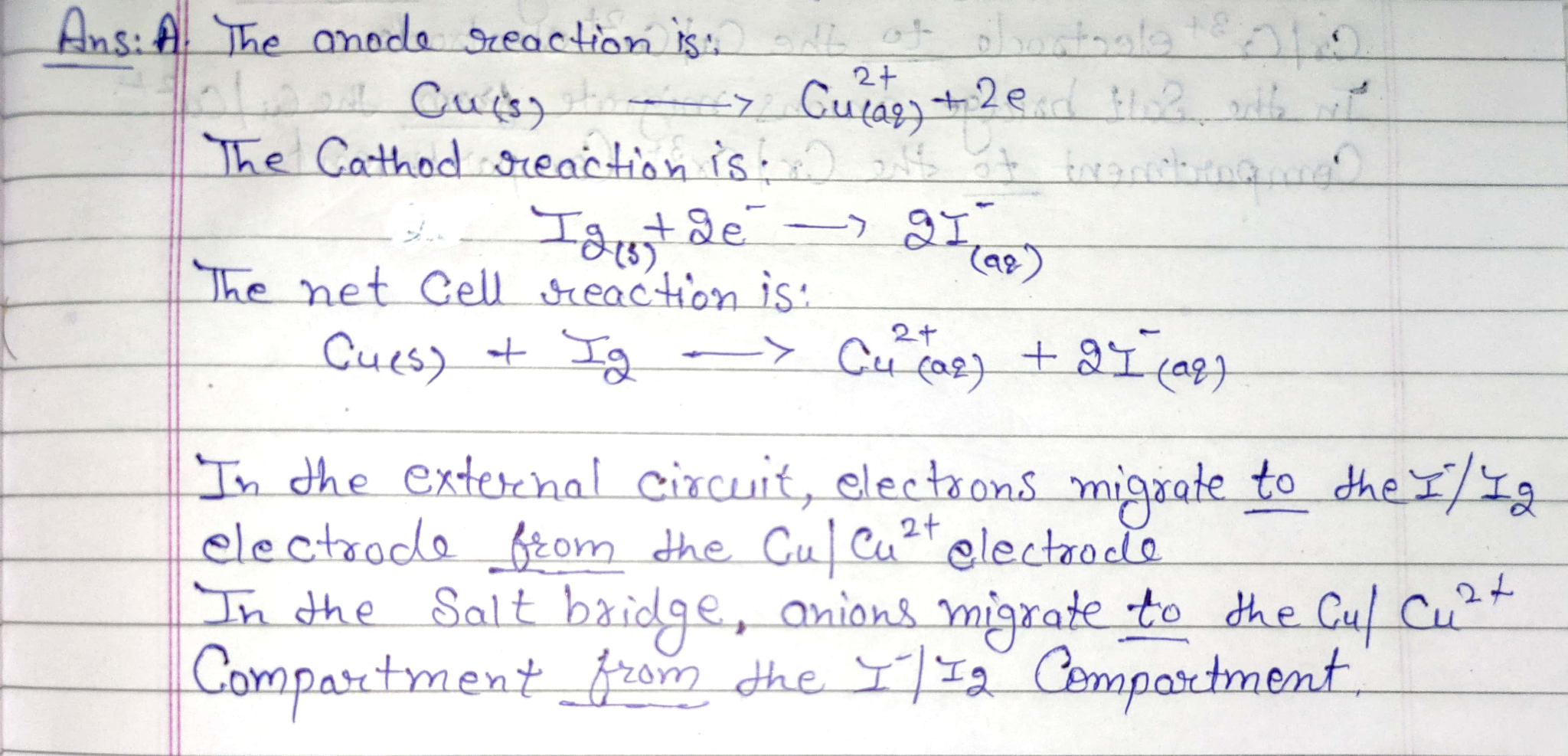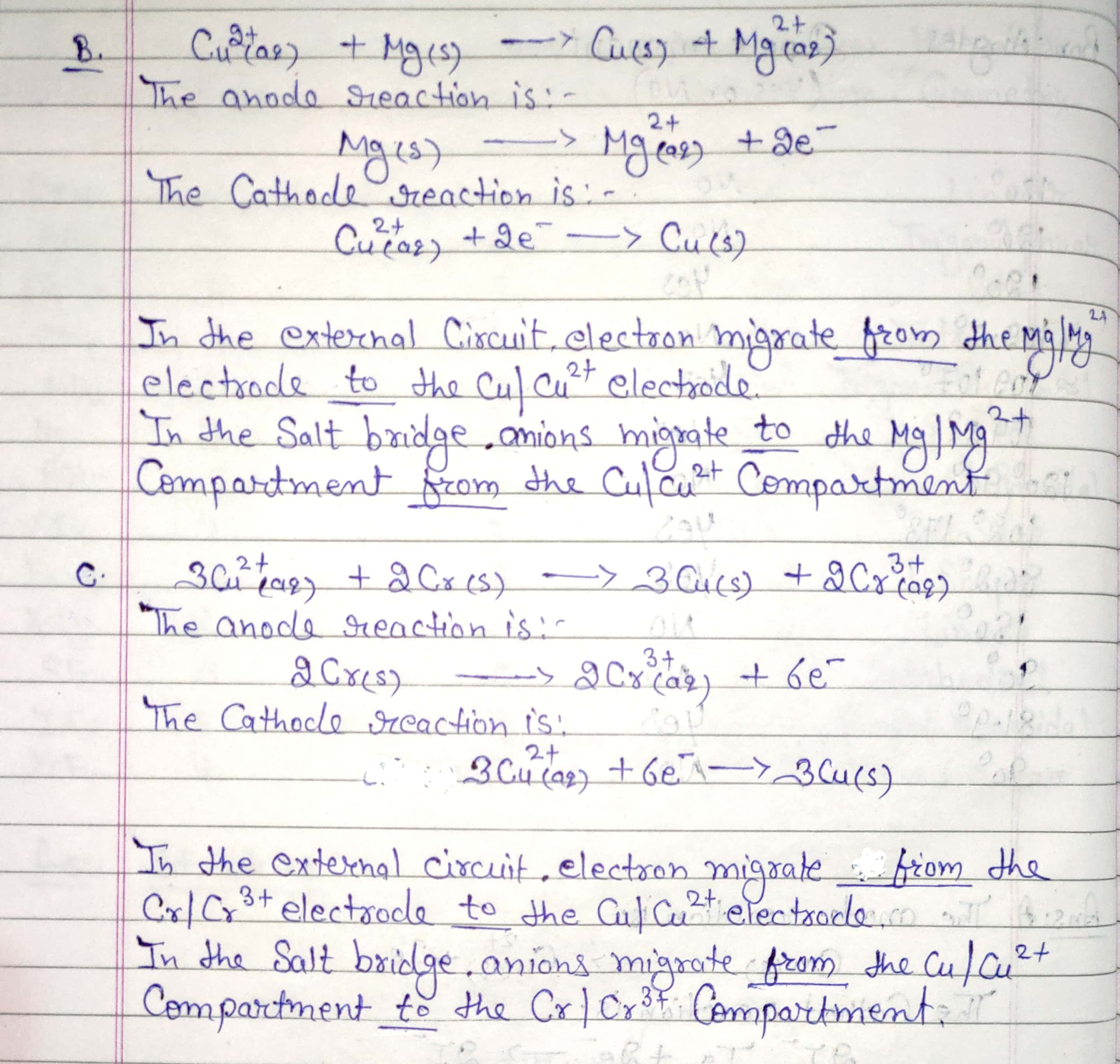#### Earn Coin

Coins can be redeemed for fabulous gifts.

Similar Homework Help Questions
• ### Enter electrons as e. A voltaic cell is constructed in which the anode is a Cr...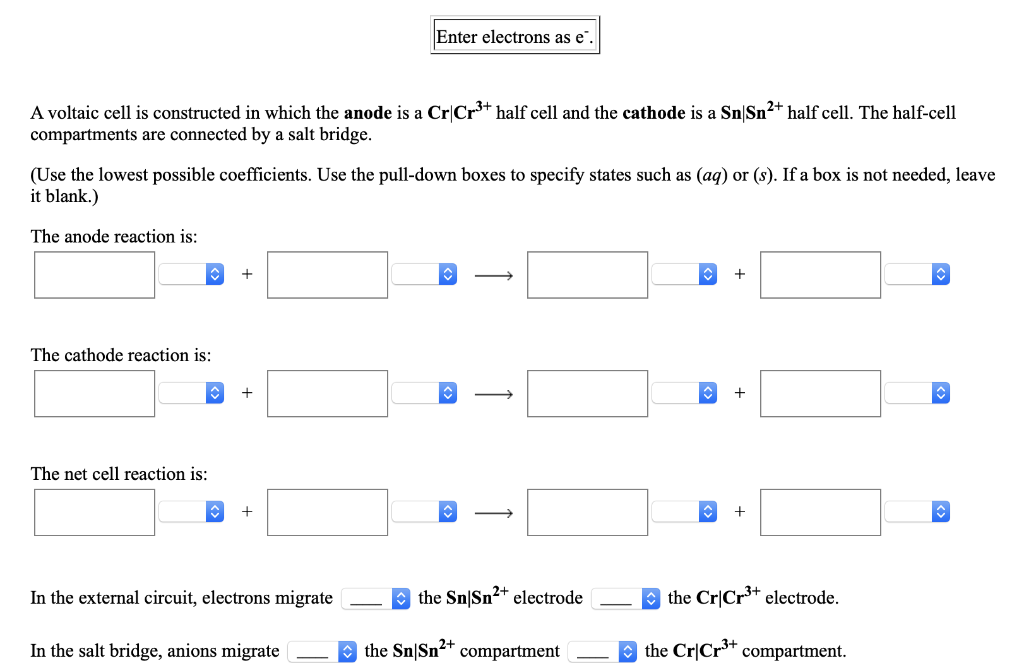Enter electrons as e. A voltaic cell is constructed in which the anode is a Cr Cr3+ half cell and the cathode is a SnSn2+ half cell. The half-cell compartments are connected by a salt bridge. (Use the lowest possible coefficients. Use the pull-down boxes to specify states such as (aq) or (s). If a box is not needed, leave it blank.) The anode reaction is: The cathode reaction is: The net cell reaction is: + + In the external...

• ### Enter electrons as e A voltaic cell is constructed in which the anode is a Mg...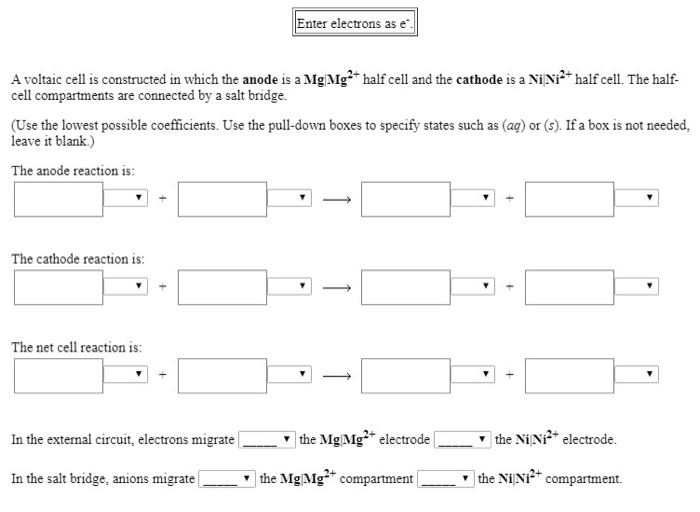Enter electrons as e A voltaic cell is constructed in which the anode is a Mg Mg2+ half cell and the cathode is a Ni Ni2+ half cell. The half- cell compartments are connected by a salt bridge. (Use the lowest possible coefficients. Use the pull-down boxes to specify states such as (ag) or (s). If a box is not needed, leave it blank.) The anode reaction is: The cathode reaction is: The net cell reaction is: In the external...

• ### Enter electrons as e A voltaic cell is constructed in which the anode is a Mn...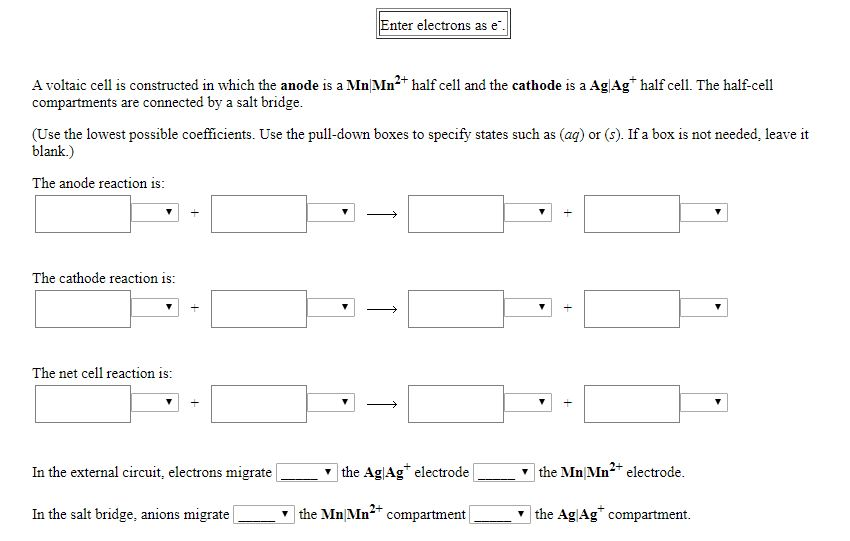Enter electrons as e A voltaic cell is constructed in which the anode is a Mn Mn+ half cell and the cathode is a Ag Agt half cell. The half-cell compartments are connected by a salt bridge. (Use the lowest possible coefficients. Use the pull-down boxes to specify states such as (aq) or (s). If a box is not needed, leave it blank.) The anode reaction is: The cathode reaction is: The net cell reaction is: In the external circuit,...

• ### Enter electrons as e A voltaic cell is constructed in which the anode is a Cr|Cr3+half cell and t...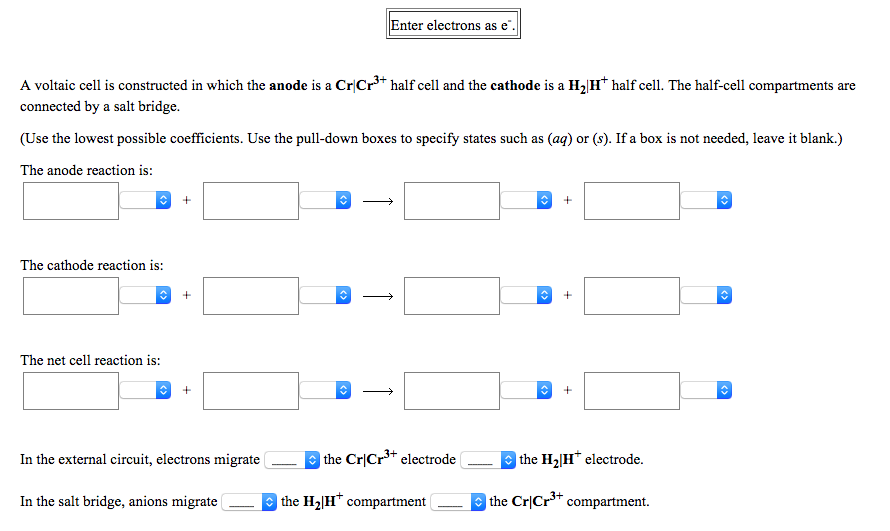Enter electrons as e A voltaic cell is constructed in which the anode is a Cr|Cr3+half cell and the cathode is a H2Ht half cell. The half-cell compartments are connected by a salt bridge (Use the lowest possible coefficients. Use the pull-down boxes to specify states such as (aq) or (s). If a box is not needed, leave it blank.) The anode reaction is: The cathode reaction is: The net cell reaction is: In the external circuit, electrons migratethe Cr(Cr3+...

• ### Enter electrons as e A voltaic cell is constructed in which the anode is a Ni...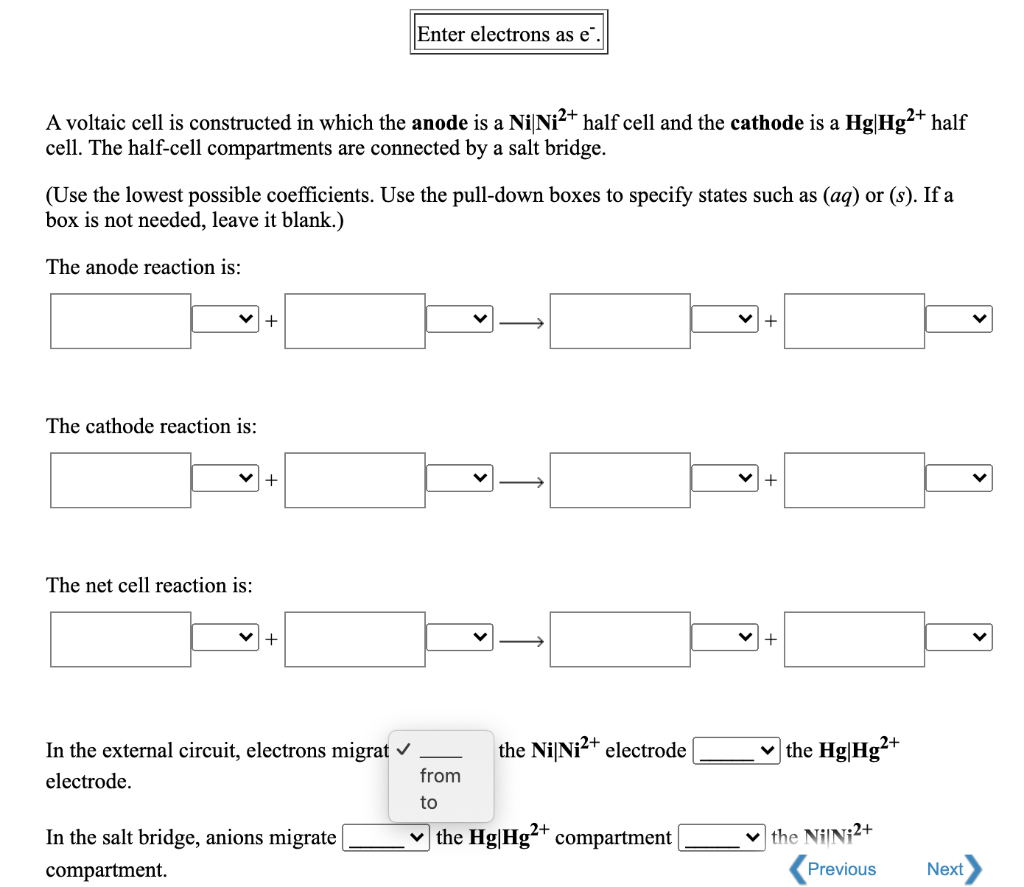Enter electrons as e A voltaic cell is constructed in which the anode is a Ni Ni2+ half cell and the cathode is a Hg|Hg2+ half cell. The half-cell compartments are connected by a salt bridge. (Use the lowest possible coefficients. Use the pull-down boxes to specify states such as (aq) or (s). If a box is not needed, leave it blank.) The anode reaction is: + + The cathode reaction is: + V> + The net cell reaction is:...

• ### A voltaic cell is constructed in which the anode is a CoCo2+ half cell and the...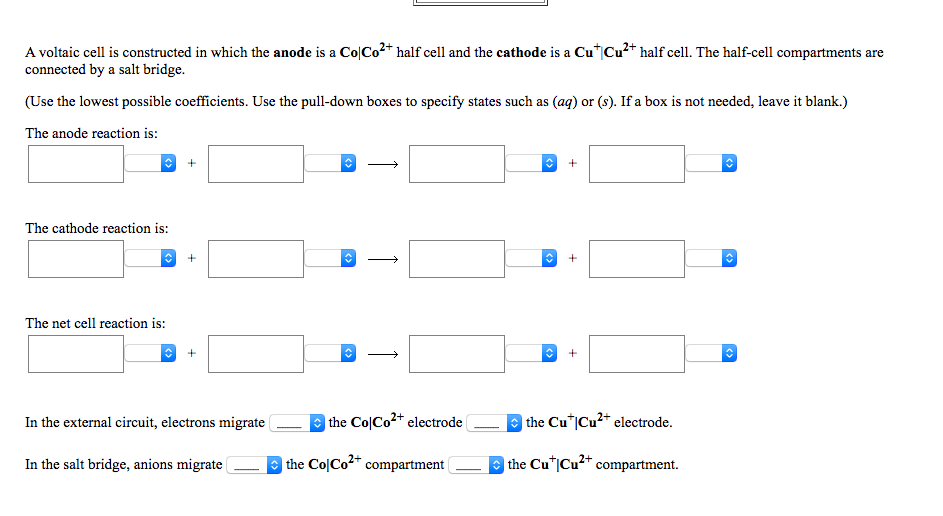A voltaic cell is constructed in which the anode is a CoCo2+ half cell and the cathode is a CuCu2+ half cell. The half-cell compartments are connected by a salt bridge. (Use the lowest possible coefficients. Use the pull-down boxes to specify states such as (aq) or (s). If a box is not needed, leave it blank.) The anode reaction is: The cathode reaction is: The net cell reaction is: In the external circuit, electrons migrate the CoCo2+ electrode —...

• ### A voltaic cell is constructed in which the anode is a Ag Ag half cell and...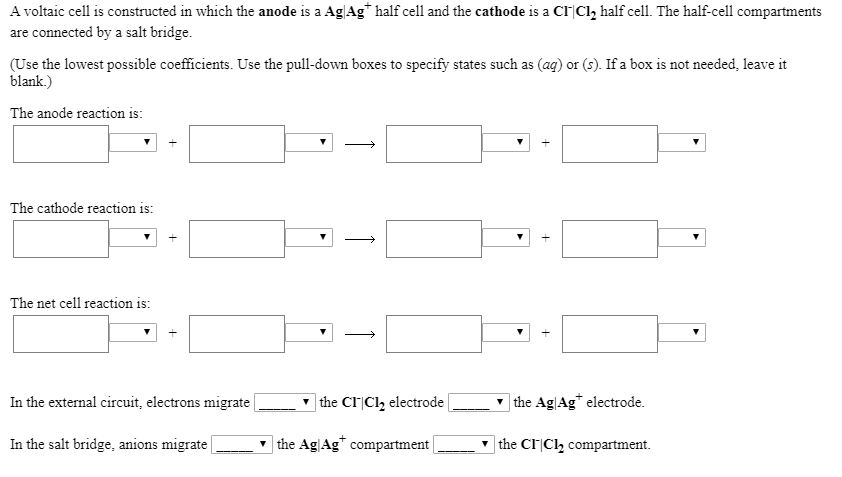A voltaic cell is constructed in which the anode is a Ag Ag half cell and the cathode is a CI Cl2 half cell. The half-cell compartments are connected by a salt bridge. (Use the lowest possible coefficients. Use the pull-down boxes to specify states such as (aq) or (s). If a box is not needed, leave it blank.) The anode reaction is: The cathode reaction is: The net cell reaction is: the Ag Ag electrode. |the CIICl2 electrode In...

• ### Enter electrons as e A voltaic cell is constructed in which the anode is a ATAN...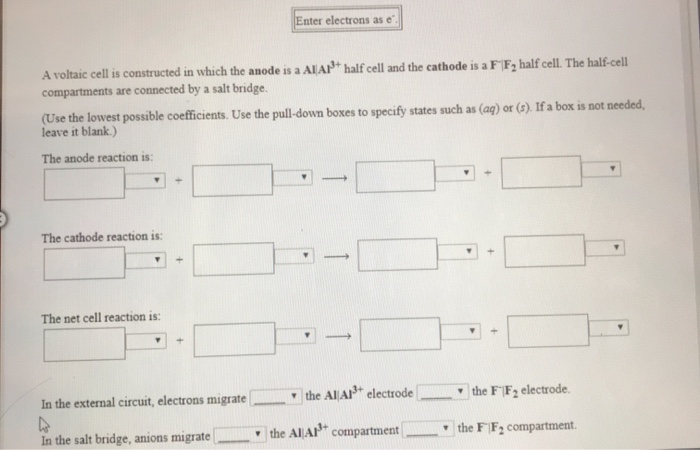Enter electrons as e A voltaic cell is constructed in which the anode is a ATAN half cell and the cathode is a FF, half cell. The half-cell compartments are connected by a salt bridge. (Use the lowest possible coefficients. Use the pull-down boxes to specify states such as (aq) or (s). If a box is not needed, leave it blank.) The anode reaction is The cathode reaction is: The net cell reaction is: In the external circuit, electrons migrate...

• ### Enter electrons as e A voltaic cell is constructed in which the anode is a Sn...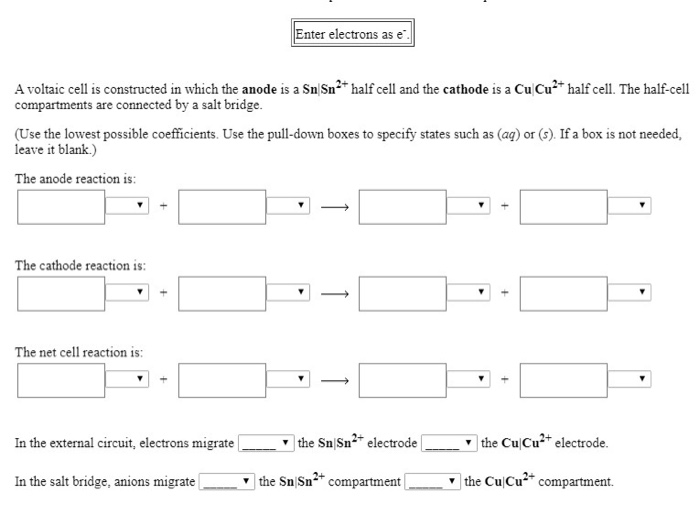Enter electrons as e A voltaic cell is constructed in which the anode is a Sn Sn2 half cell and the cathode is a Cu Cu compartments are connected by a salt bridge. half cell. The half-cell (Use the lowest possible coefficients. Use the pull-down boxes to specify states such as (aq) or (s). If a box is not needed, leave it blank) The anode reaction is: The cathode reaction is: The net cell reaction is: the Sn Sn2 electrode...

• ### A) B) C) Enter electrons as e. Use smallest possible integer coefficients for ALL reactions. If...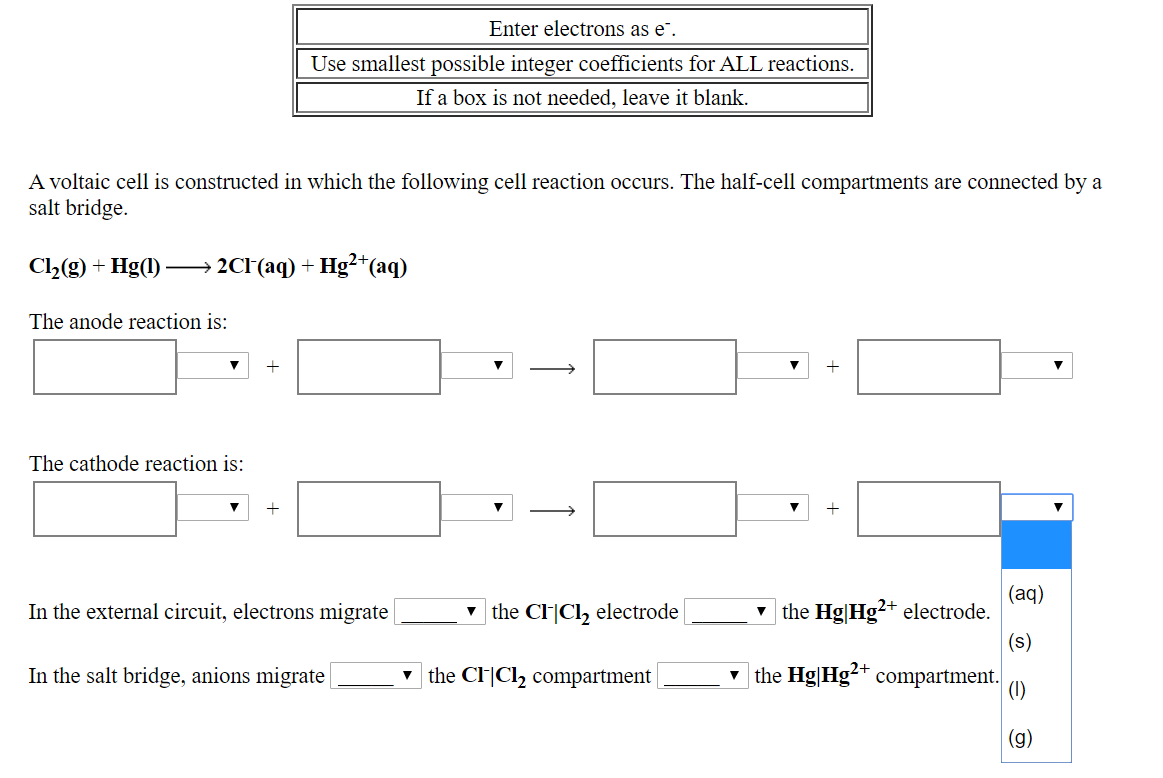A) B) C) Enter electrons as e. Use smallest possible integer coefficients for ALL reactions. If a box is not needed, leave it blank. A voltaic cell is constructed in which the following cell reaction occurs. The half-cell compartments are connected by a salt bridge. Cl2(g) + Hg(1) —→ 2CH(aq) + Hg2+(aq) The anode reaction is: The cathode reaction is: In the external circuit, electrons migrate the C-Cl, electrode | y the Hg|Hg2+ electrode. In the salt bridge, anions migrate...